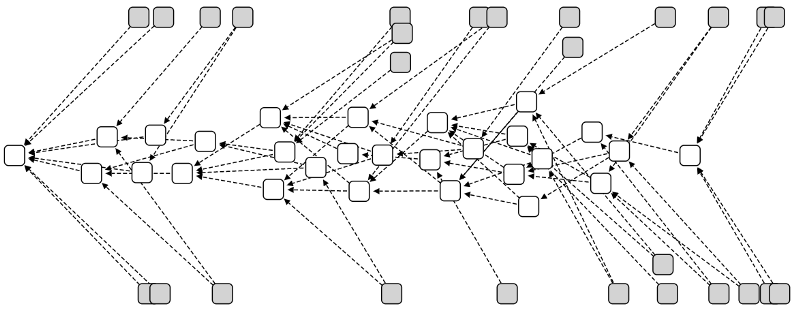# Alpha: playing with randomness

If you have been following the Illustrated Introduction to the Tangle series, you might remember a mysterious parameter called α, which affects the level of randomness in the random walk. In this article we will go into the specific way in which α affects tip selection, and mention some issues to consider when writing a software implementation.

Note that this article assumes a basic understanding of how the Tangle is built, and in particular what approvers and cumulative weights are. You should also be comfortable with the exponential function, and have some understanding of probability theory.

### Why do we need randomness again?

To set the stage for understanding α, we need to remember why we perform a random walk in the first place. The context is tip selection: each new transaction must approve two previous transactions. The way this choice is made is crucial in determining how the Tangle looks and behaves.

The tip selection method should ideally provide the following two features:

1. Once a transaction accumulates a large number of approvers, it is very unlikely to get abandoned.
2. Honest transactions should get approved quickly.

To achieve the first goal, we might decide to perform a deterministic walk from the genesis to the tips, always going towards the approver with the largest cumulative weight. However, this would hurt the second goal: only a single central chain would get approvals, while most transactions would be left behind.When we choose tips by always going towards the heaviest approver, many transactions are left behind.

In order to reach a compromise between these two goals, we introduce some randomness. We prefer the heavier approvers, but still give some probability to lighter ones as well.

### Mathematical definition

We are defining a transition function, that tells us the probability to go from approvee to approver during the random walk. We would like this probability to be large for approvers with a high cumulative weight, and small for lighter approvers.

The transition function we use is defined as follows:

Where Pxy is the probability to walk from x to y, Hy is the cumulative weight of transaction y, and z ~> x means “z directly approves x”.

In other words, the probability of walking from x to y increases exponentially with the cumulative weight of y, multiplied by α. The sum in the denominator is a normalization factor, which sets the total transition probabilities sum to one.

### Example

In the following example, the random walk reached transaction X, which has three approvers: A, B, and C. In order to figure out the transition probabilities, we first have to calculate the cumulative weights. A has a single approver, and therefore cumulative weight 2. B has two approvers, so it has weight 3. Finally, C has no approvers, so its weight is 1.

Let’s start with α=1, and plug the numbers into the formula above:

We can see that C has a much smaller probability to get chosen than A or B, since its weight is smaller.

If we set α to a smaller value, we decrease the bias against C. For example, for α=0.1, we get the following probabilities:

There is still a slight bias against transaction C, but it’s far less pronounced.

If we examine the extreme scenario of α=0, all approvers are completely equivalent, and have a probability of 1/3. This is the case where the weights stop mattering, and the walk is completely random.

On the other extreme, we have the case of a very large alpha. In this scenario, the probability to walk towards A is 1, and B and C have transition probability zero. This case is analogous to a blockchain: we only approve the single most likely tip, and never merge different branches together.

### Summary

This article was a bit more mathematical than the previous ones, and hopefully you managed to follow along. As always, you are very welcome to ask questions, either below in the responses or in #tanglemath on our Discord.